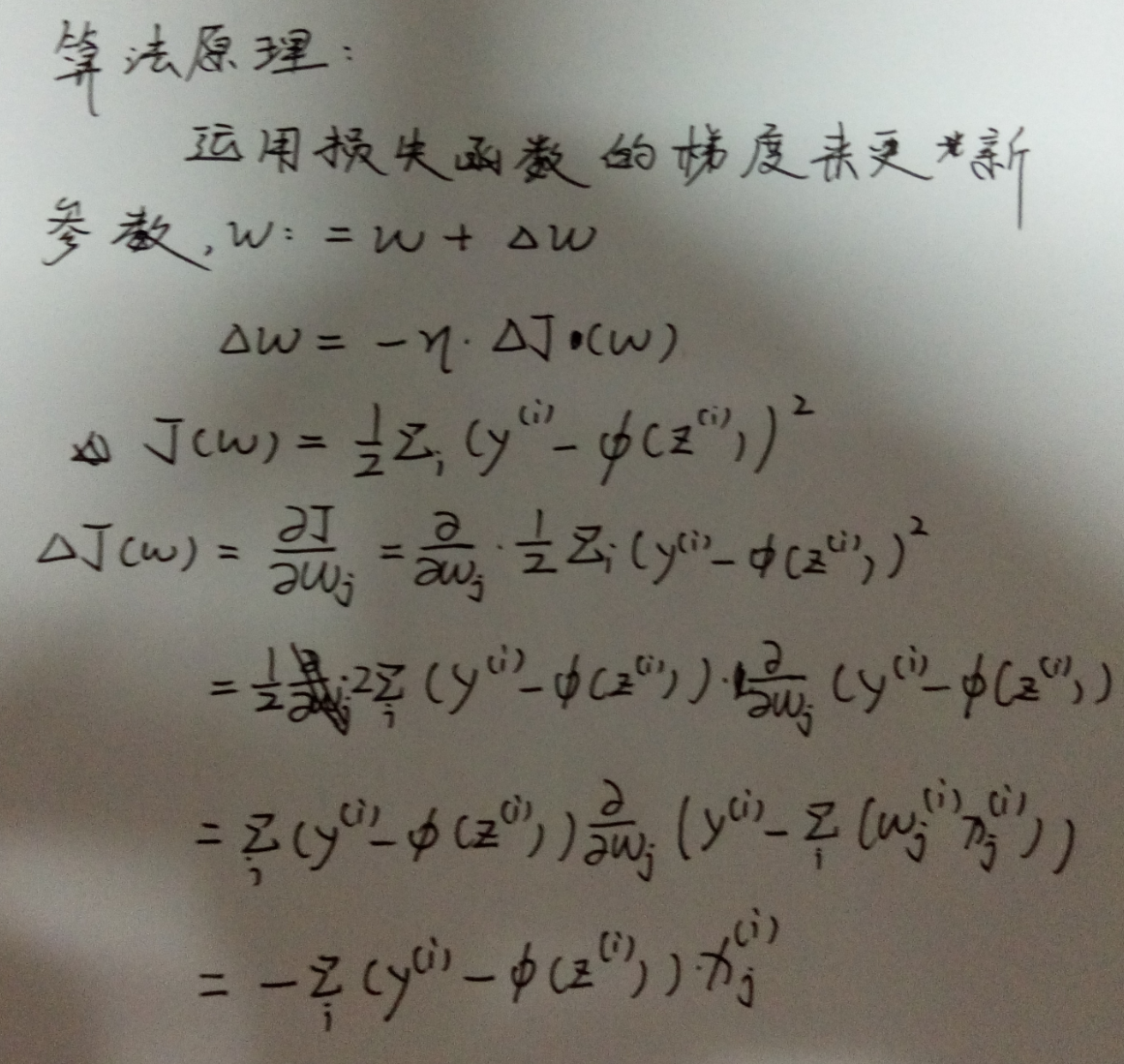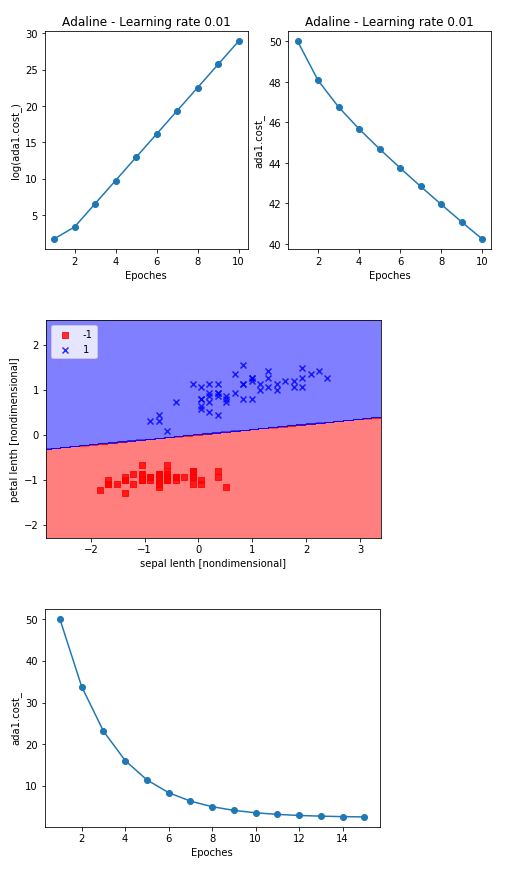## Python实现梯度下降法及算例分析以及可视化_Airuio的博客-程序员宅基地#实现梯度下降法：
import numpy as np
def __init__(self,eta=0.01,n_iter=50):  #定义超参数学习率和迭代次数
self.eta = eta
self.n_iter = n_iter

def fit(self,X,y):        #定义权重系数w和损失函数cost
self.w_ = np.zeros(1+X.shape)
self.cost_ = []

for i in range(self.n_iter):                   #更新权重
output = self.net_input(X)            #计算预测值
errors = (y - output)                     #统计误差
self.w_[1:] += self.eta*X.T.dot(errors)
self.w_ += self.eta * errors.sum()
cost = (errors**2).sum()/2.0          #损失函数
self.cost_.append(cost)
return self

def net_input(self,X):
return np.dot(X,self.w_[1:]) + self.w_

def activation(self,X):
return self.net_input(X)

def predict(self, X):

return np.where(self.activation(X) >= 0.0, 1, -1)

import numpy as np
import pandas as pd
import matplotlib.pyplot as plt
from matplotlib.colors import ListedColormap

y = df.iloc[0:100,4].values            #取前100列数据，4列为标识
y = np.where(y == 'Iris-setosa', -1,1)
X = df.iloc[0:100,[0,2]].values      #iloc为选取表格区域，此处取二维特征进行分类,values为返回不含索引的表

plt.scatter(X[:50,0],X[0:50,1],color = 'red',marker = 'o', label = 'setosa')
plt.scatter(X[50:100,0],X[50:100,1],color = 'blue',marker = 'x', label = 'versicolor')
plt.xlabel('petal lenth')
plt.ylabel('sepal lenth')
plt.legend(loc = 2)    #画出标签以及标签的位置参数
plt.show()             #出图
#以上六行与分类无关，仅仅是为了直观的感受两块数据的分布区域

fig,ax = plt.subplots(nrows = 1 , ncols = 2, figsize = (8,4))
'''

plt.subplots(nrows = 1 , ncols = 2, figsize = (8,4)中nrows表示几行图，ncols表示几列
figsize为图片大小。
'''
ax.set_xlabel('Epoches')
ax.set_xlabel('Epoches')
plt.show()
'''

（原值-均值）/标准差，可以用numpy中的mean和std方法便捷的获得
'''
X_std = np.copy(X)                #将样本特征归一化、标准化
X_std[:,0] = (X[:,0] - X[:,0].mean()) / X[:,0].std()
X_std[:,1] = (X[:,1] - X[:,1].mean()) / X[:,1].std()

def plot_decision_region(X,y,classifier,resolution = 0.02):
markers = ('s','x','o','~','v')
colors = ('red','blue','lightgreen','gray','cyan')
cmap = ListedColormap(colors[:len(np.unique(y))])

#画出界面
x1_min, x1max = X[:,0].min() - 1, X[:,0].max() + 1
x2_min, x2max = X[:,1].min() - 1, X[:,1].max() + 1

xx1,xx2 = np.meshgrid(np.arange(x1_min,x1max,resolution),

np.arange(x2_min,x2max,resolution))   #生成均匀网格点，

'''

meshgrid的作用是根据传入的两个一维数组参数生成两个数组元素的列表。如果第一个参数是xarray，    维度是xdimesion，第二个参数是yarray，维度是ydimesion。那么生成的第一个二维数组是以xarray为行，ydimesion行的向量；而第二个二维数组是以yarray的转置为列，xdimesion列的向量。

'''

Z = classifier.predict(X = np.array([xx1.ravel(),xx2.ravel()]).T)
Z = Z.reshape(xx1.shape)
#在全图上每一个点（间隔0.2）计算预测值，并返回1或-1

plt.contourf(xx1,xx2,Z,alpha = 0.5,cmap = cmap) #画出等高线并填充颜色
plt.xlim(xx1.min(),xx1.max())
plt.ylim(xx2.min(),xx2.max())

#画上分类后的样本
for idx,cl in enumerate(np.unique(y)):
plt.scatter(x=X[y==cl,0], y=X[y==cl,1],alpha=0.8,
c=cmap(idx),marker=markers[idx],label=cl)

plot_decision_region(X_std, y, classifier = ada)    #展示分类结果
plt.xlabel('sepal lenth [nondimensional]')
plt.ylabel('petal lenth [nondimensional]')
plt.legend(loc = 2)
plt.show()

plt.xlabel('Epoches')

plt.show()### ruby 中ObjectSpace.define_finalizer 遇到的问题_ruby finalize-程序员宅基地

ObjectSpace.define_finalizer 方法可以接受一个 proc, 当某个对象被 GC 销毁时会回调该 proc. 当我按照文档使用这个方法的时候却发现一个很奇怪的现象, 测试代码如下:def foo str = 'hello, world' * 1_000_000 ObjectSpace.define_finalizer(str, proc { |id|_ruby finalize

### pycuda 之 安装与简单使用-程序员宅基地

pycuda安装： （1）查看CUDA版本：cat /usr/local/cuda/version.txt （目前实验CUDA版本为：CUDA Version 9.0.176） （2）查看cudnn版本：cat /usr/local/cuda/include/cudnn.h | grep CUDNN_MAJOR -A 2 （目前实验cudnn版本：7.0） ..._pycuda